# Tetravalency of carbon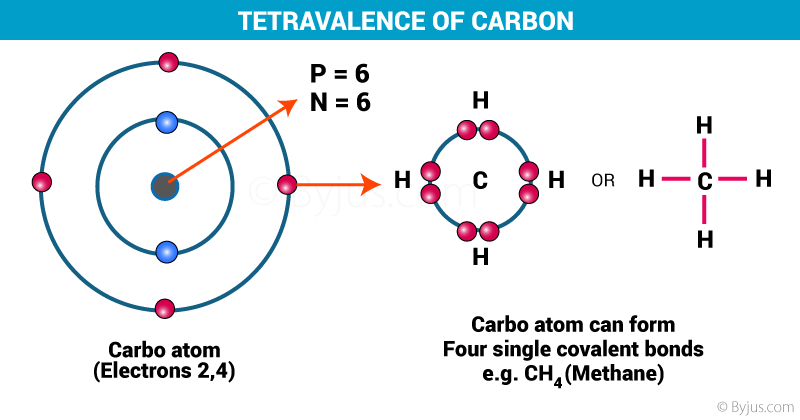The valency of carbon is 4 and it is, therefore, said to be tetravalent

The branch of organic chemistry deals with the compounds of carbon. Carbon has a valency of 4 and is just one element in the list of over a hundred elements in the periodic table. Due to the specific properties of the element, Carbon has established its importance among the other elements. The properties which make carbon so important are:

1. Catenation
2. Tetravalency
3. Size of a carbon atom

Although there is no specific definition, the compounds in solid, liquid or gaseous state which contain covalently bonded carbon atoms in their molecules are known as organic compounds. Let us see how the tetravalent nature of carbon affects carbon compounds.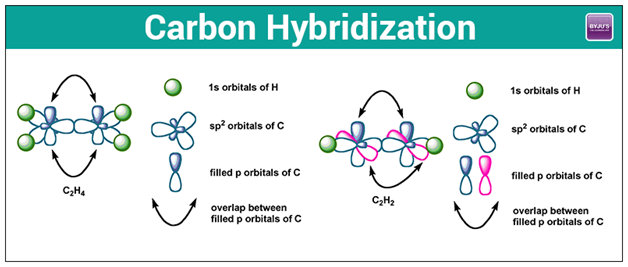Hybridization of Carbon

## Hybridization of Carbon

Hybridization is the concept of combining atomic orbitals to make new hybrid orbitals appropriate to represent their properties of bonding. Hybridized orbitals are helpful in describing the shape of molecular orbitals apart from being a major part of valence bond theory.

The name hybrid applies to the atomic orbitals that contribute to the hybridization. For example, in methane whose chemical formula is CH4, a set of sp3 orbitals develops by combining one s-orbital and three p-orbitals on the carbon atom. These orbitals direct towards the four hydrogen atoms placed at the vertices of a regular tetrahedron.

Ethene(C2H4) consists of a double bond between the carbon atoms. Here, the carbon hybridizes by sp2. In sp2 hybridization, the 2s orbital mingles with two among the three 2p orbitals available, making total 3 sp2 orbitals with one remaining p-orbital. In ethane, two atoms of carbon develop a sigma bond by overlaying two sp2 orbitals, where every carbon atom makes two covalent bonds with hydrogen by overlapping all s-sp2 with 120o angles. The pi bond among the carbon atoms develops by a 2p-2p overlap. The hydrogen-carbon bonds have equal length and strength that satisfies with experimental proof.

Many bonds also exist between non-similar atoms. When two atoms of oxygen are brought near opposite sides of the carbon atom in CO2, one among the p orbitals on every oxygen makes a pi bond with anyone among the p-orbitals of carbon. Here, the sp hybridization forms two double bonds.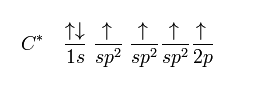### sp2 hybridization

The sp2 carbon hybridizes in ethene since one π bond is needed for the double bond among the carbons and three σ bonds made for every carbon atom.

## Tetravalence of Carbon

Ground state electronic configuration of carbon is 1s2, 2s2, 2p2. It has 4 valence electrons, so the probability of formation of four bonds is maximum. The bonds formed by the s orbital electrons will not be the same as that of p orbital electrons. So in the formation of one molecule of CH4, there will be a combination of 1 C atom with 4 H atoms.

The following types of bonds can be formed: C(s)-H(s), C(s)-H(s), C (p)-H(s) and C (p)-H(s). Out of the four bonds, we have two ‘directional’ C (p)-H(s) and two non-directional bond C(s)-H(s). (Note: As we know that s orbitals are spherical in shape and do not have any specific direction and p orbitals have shapes in three directions x, y, and z-axis.) The strength of the bond will also differ as C (p)-H(s) bond will be less stronger than the C(s)-H(s) bond as s overlapping is stronger.

But practically all the bonds of CH4 are identical. This creates a problem. To solve this problem hybridization theory has been stated. It is mainly a concept in which atomic orbitals are mixed with new hybrid orbitals which are most suited for the pairing of electrons to form chemical bonds.  It can be understood by the fig given below: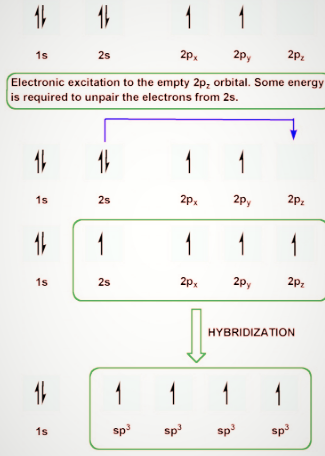An Electron jumps from the 2s orbital to the 2p orbital, resulting in four unpaired electrons

In the fig, three p and one s-orbital are hybridized to give four identical sp3 hybridized orbitals. In a similar manner, we can also get sp and sp2 hybridization. The only change will be that sp2 will have only two p orbitals. Now from the VSEPR theory, we know that sp and sp2 hybridized molecules are planar in structure. Whereas sp3 hybridized molecules take tetrahedral shape to become more stable (this structure leads to minimum energy state).

The angle between each carbon and hydrogen atom is 109.5  in tetrahedral geometry, 120  in sp2 and 180  in sp hybridization.  So methane will have sp3 hybridization having a tetrahedral shape. Whereas examples for sp and sp2 hybridization are acetylene and ethene respectively that are planar in shape. Now we can clearly understand that ethane will have two unhybridized p orbitals from each sp2 carbon which will overlap with each other to form a pi bond. So by this, we can infer that double bond is just a combination of two single bonds. The figure given below demonstrates the hybridization in the carbon atoms of ethylene and acetylene.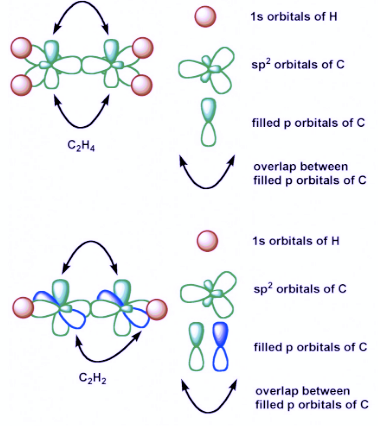Hybridization of Carbon in Ethylene and Acetylene

This article was a brief description of the tetravalent nature of carbon and how it affects the shape of carbon molecules. To know more about organic compounds, the anomalous behaviour of carbon, download Byju’s The Learning App.